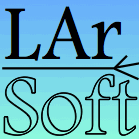# LArSoftSoftware for Liquid Argon time projection chambers

View My GitHub Profile

# Guidelines on using the geometry

Detailed information is available on the Geometry Package and at important concepts

• always use complete ID classes to identify a part of the detector; for example, identify a plane with a `geo::PlaneID`, that also includes information about the cryostat and TPC the plane belongs to, rather than the simple plane index in the TPC; complete ID types are defined in `SimpleTypesAndConstants/geo_types.h` in `larcore`
• use geometry methods that have geometry IDs as arguments: prefer `geo::GeometryCore::Nplanes(geo::TPCID const&amp;)` to `geo::GeometryCore::Nplanes()`, as the latter will use a very specific TPC, and the choice of which one is beyond your control
• when iterating through geometry objects, use the geometry iterators from `geo::GeometryCore`; some more advanced iterations haven’t been implemented yet simply for lack of request, but they can be implemented on demand (e.g. iterate on all the wires in a given TPC)
• there is seldom need to “decay” a geometry ID into an index: e.g., a vector keyed by plane index (that should be, incidentally, the type `geo::PlaneID::PlaneID_t`), `std::vector<DataClass>`, may be replaced by a `std::map<geo::PlaneID, DataClass>`
• where a vector is the only reasonable choice, have the parent ID travel with it; in the above example, store a `geo::TPCID` together with the vector, so that reconstituting the original ID is easy
• always query `geo::GeometryCore` for geometry information; e.g.,
• do not assume there are 3 wire planes, but instead use `geo::GeometryCore::Nplanes(tpcid)` to find how many
• ask global coordinates to the `geo::TPCGeo` (using e.g. `geo::TPCGeo::MinX()`, `geo::TPCGeo::MaxX()`, etc.) rather than compute it yourself
• ask which ID the channel on the next wire has, rather than assuming it’s the next channel ID (this requires multiple passes, but `GeometryCore` can be extended to do them for you)
• always use world coordinates whenever possible
• beware of assumptions
• there is not such a thing as the TPC, but there is a TPC
• do not rely on knowing where “0” is on an axis, ask `GeometryCore` instead
• don’t assume that mappings between readout channels and wires are one-to-one
• don’t assume the wire pitch is the same on all planes (currently all wires on the same wire plane should have the same pitch, barred bugs)

Some of the steps you should consider to review your (or others’) code:

• check all the calls to `geo::Geometry` service; among other things, look for:
• calls without a full geometry ID
• calls returning a vector, of which only the first value is used
• keep track of how the geometry object IDs move around
• note if there is code that does not query `Geometry` when you expect it would: it might have geometry information cached, and in that case one should verify that they are cached correctly, or there might be assumptions
• investigate any hard coded number and where it comes from
• note if a call that can return an error state is not checked against it (e.g., calling `geo::GeometryCore::NearestWireID()` without checking if the returned ID `.isValid`)
• check the `try`/@catch@ blocks to make sure errors are correctly dealt with as well; if some code ignores an exception, it must explain why!
• any code using `geo::GeometryCore::DetectorName()` automatically triggers a red alert, as it implies detector dependency
• (there is not only geometry!) every time a… time is used, check that it uses the correct time reference (first tick, t 0) and the correct range (how many ticks?); such information can be retrieved via `TimeService` and `DetectorProperties` services

Every time you find you need to check, consider also adding a comment that explains why the core is correct as is.